# Pompeiu problem

(diff) ← Older revision | Latest revision (diff) | Newer revision → (diff)

Let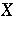be a Hausdorff topological space (cf. also Hausdorff space; Topological space),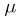a non-negative Radon measure on, anda topological group of continuous self-mappings ofleavinginvariant. For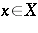and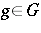,denotes the action ofon. A family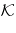of compact subsets ofis said to have the Pompeiu property if the linear mapping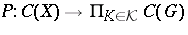given by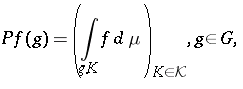(a1)

is injective.

A typical example occurs when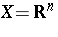,is the Lebesgue measure, andis the Euclidean group of orientation-preserving rigid motions. Let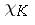denote the characteristic function of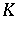and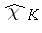its Fourier transform, which is an entire function of exponential type (cf. also Entire function) in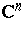. In this case one can prove [a7], [a12] thathas the Pompeiu property if and only if(a2)

Whenis the ball, (a2) can never be satisfied but if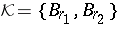, a pair of balls of radiiand(the centre plays no role in this case), then it has the Pompeiu property if and only if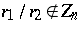, where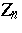is the set of fractionsfor which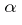and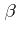are positive roots of. (Here,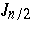is the Bessel function of the first kind and order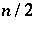, cf. Bessel functions.)

The key statement of the equivalence of the Pompeiu property with (a2) holds whenis an irreducible symmetric space of rank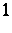and non-compact type and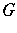is its group of orientation-preserving isometries [a12].

Whenis a bounded open set with Lipschitz boundary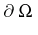insuch thatis connected, and if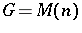, then failure of the Pompeiu property for the singleton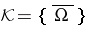is equivalent to the existence of an eigenvalue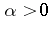for the overdetermined Neumann boundary value problem for the Euclidean Laplacian (cf. also Neumann boundary conditions)(a3)

It was shown by S. Williams [a9] that if (a3) has a solution, thenmust be real-analytic, which allows for many positive examples of the Pompeiu property. The equivalence between (a3) and the failure of Pompeiu property and Williams' observation also holds whenis a non-compact symmetric space of rankwiththe invariant Laplacian in this case [a4].

The natural conjecture that the existence of a solutionfor (a3) is equivalent tobeing a Euclidean ball is usually called Schiffer's conjecture. For instance, [a1] contains the result that for convex planar sets the existence of infinitely many eigenvalues for (a3) implies thatis a disc. This inspired work of M. Agranovsky, C.A. Berenstein and P.C. Yang, N. Garofalo and F. Segala, T. Kobayashi, and others. See the excellent bibliographic survey [a11] for the details on the progress made on this conjecture up to date (1998), as well as general background on the Pompeiu property.

For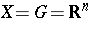with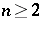, condition (a2) is only known to be necessary, due to the failure of the spectral synthesis, [a7]. For instance, for, except for elementary examples of the type of three squares with sides parallel to the axes and sizes,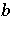,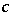none of whose quotients is rational, one can show that if one takes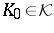to be, e.g., a rectangle, then (a2) is also sufficient for the Pompeiu property [a6].

This case of the Pompeiu problem has many applications in image and signal processing and leads to the problem of deconvolution, that is, given a finite family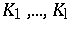, find distributions of compact supportsuch that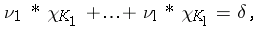which amounts to finding a left inverse of the Pompeiu mapping (a1). See [a3], [a5], [a8] for details.

There is a local variant of the Pompeiu problem. For instance, letbe the unit ball of, letbe a pair of balls centred at the origin such that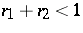and, then the values of all the integrals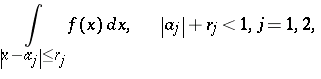are enough to determine any function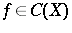(the Berenstein–Gay theorem); see [a2], [a10], [a11], for additional references, extensions, as well as relations to the deconvolution problem mentioned earlier.